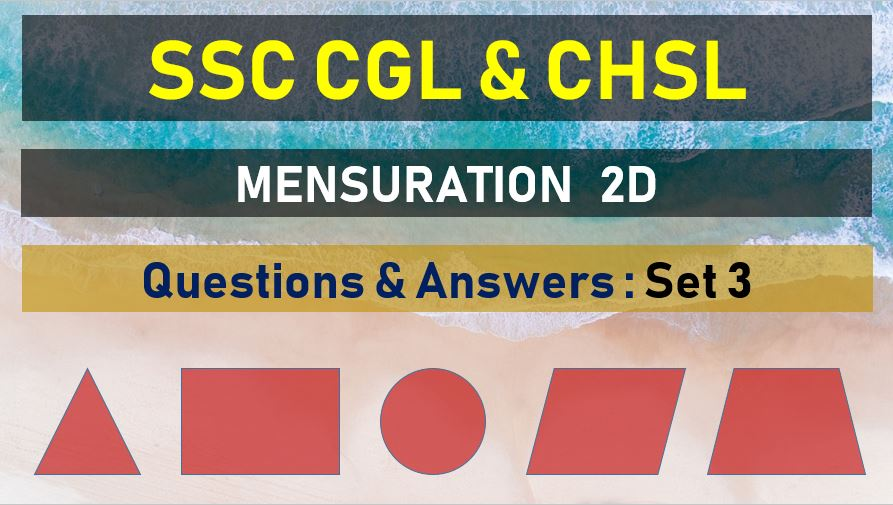Friday, May 7, 2021
Home > mensuration > SSC CGL Mensuration 2D Questions Answers Set 3

# SSC CGL Mensuration 2D Questions Answers Set 3SSC Mensurations 2D
Area and Perimeter Questions Sets
Set 1Set 2Set 3Set 4Set 5
Set 6Set 7Set 8Set 9Set 10

SSC CGL Mensuration 2D Questions Answers Set 3: Ques No 1

The perimeter of five squares are 24 cm, 32 cm, 40 cm, 76 cm and 80 cm respectively the perimeter of another square equal in area to sum of the areas of these squares is

Options:

A. 31 cm

B. 62 cm

C. 124 cm

D. 961 cm

Solution:

SSC CGL Mensuration 2D Questions Answer Set 3: Ques No 2

There is a rectangular tank of length 180 m and breadth 120 m in a circular field of the area of the land portion of the field is 40000 m2, what is the radius of the field ?

Options:

A. 130 m

B. 135 m

C. 140 m

D. 145 m

Solution:

SSC CGL Mensuration 2D Questions Answers Set 3: Ques No 3

The length of a rectangular hall is 5m more than its breadth. The area of the hall is 750m2. The length of the hall is

Options:

A. 15 m

B. 22.5 m

C. 25 m

D. 30 m

Solution:

SSC CGL Mensuration 2D Question Answers Set 3: Ques No 4

If the length and breadth of a rectangle are in the same ratio 3:2 and its perimeter is 20 cm, then the area of the rectangle (in cm3 ) is

Options:

A. 24 cm2

B. 36 cm2

C. 48 cm2

D. 12 cm2

Solution:

SSC CGL Mensuration 2D Questions Answers Set 3: Ques No 5

The perimeter of a rectangle and a square are 160 m each. The area of the rectangle is less than that of the square by 100 sq. m. The length of the rectangle is

Options:

A. 30 m

B. 60 m

C. 40 m

D. 50 m

Solution:

SSC CGL Mensuration 2D Questions Answer Set 3: Ques No 6

A path of uniform width runs round the inside of a rectangular field 38 m long and 32 m wide , if the path occupies 600m2, then the width of the path is

Options:

A. 30 m

B. 5 m

C. 18.75 m

D. 10 m

Solution:

SSC CGL Mensuration 2D Questions Answers Set 3: Ques No 7

The perimeter of the floor of a room is 18 m. What is the area of the walls of the room if the height of the room is 3 m?

Options:

A. 21 m2

B. 42 m2

C. 54 m2

D. 108 m2

Solution:

SSC CGL Mensuration 2D Questions Answer Set 3: Ques No 8

A copper wire is bent in the shape of a square of area 81 cm2. If the same wire is bent in the form of a semicircle, the radius ( in cm) of the semicircle is (take pie = 22/7)

Options:

A. 126

B. 14

C. 10

D. 7

Solution:

SSC CGL Mensuration 2D Questions Answers Set 3: Ques No 9

A copper wire is bent in the form of a square with an area of 121 cm2. if the same wire is bent in the form of a circle , the radius ( in cm) of the circle is (take pie = 22/7)

Options:

A. 7

B. 14

C. 8

D. 12

Solution:

SSC CGL Mensuration 2D Question Answers Set 3: Ques No 10

A street of width 10 meters surrounds from outside a rectangular garden whose measurement is 200 m x 180 m. the area of the path (in square meters) is

Options:

A. 8000

B. 7000

C. 7500

D. 8200